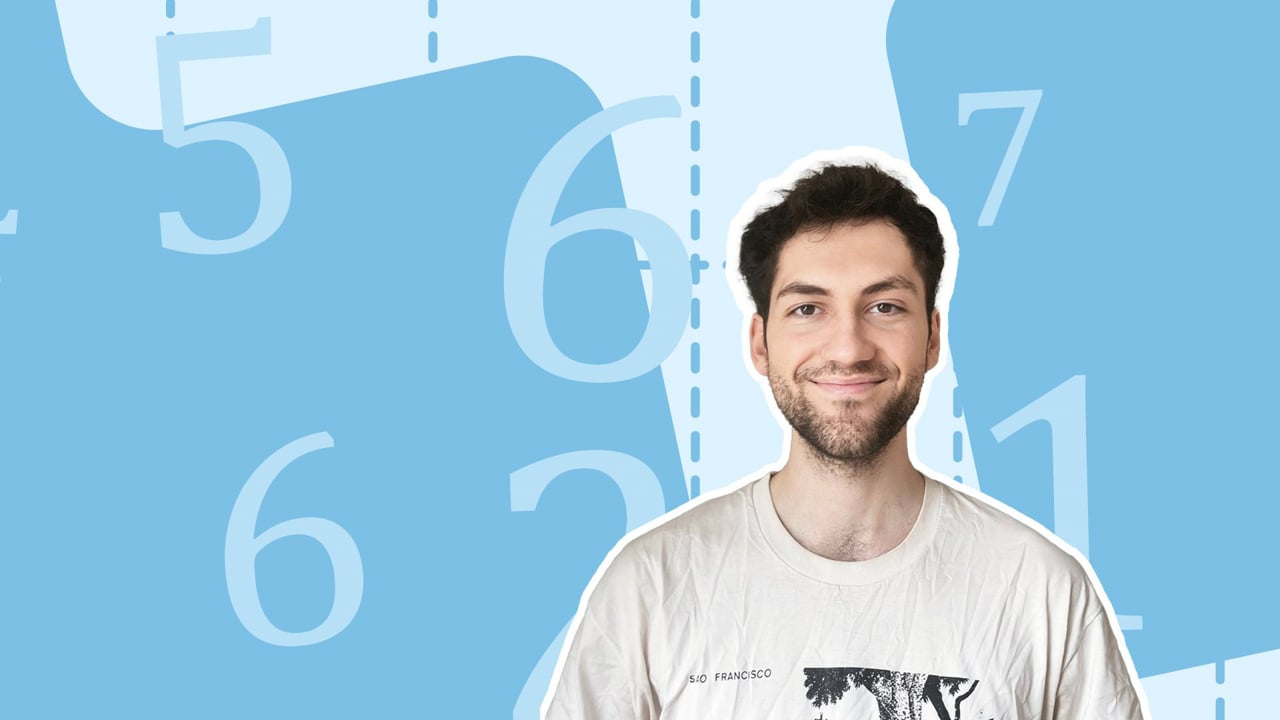Chapter OverviewMaths

Number and place value

Multiplication and division

Fractions

Measurement

Geometry - properties of shapes

Geometry - position and direction

Statistics

Maths

# Multiplying fractions and mixed numbers0%

Summary

# Multiplying fractions and mixed numbers

## In a nutshell

Fractions and mixed numbers can be multiplied by whole numbers.

##### Example 1

At a restaurant three people choose pizza from the menu.  Each person eats $\frac{3}{4}$ of a pizza.  How much pizza do they eat altogether?Three lots of three quarters totals nine quarters.

$\frac{3}{4}\times3 = \underline{\frac{9}{4}}$​​

## Multiplying fractions by whole numbers

#### procedure

 1 Multiply the numerator of the fraction by the whole number. Note: The denominator does not change. 2 Simplify the fraction if possible.

##### Example 2

Multiply $\frac{3}{12}$ by $3$.  Give your answer as a fraction in its simplest form.

Multiply the numerator of the fraction by the whole number:

$\frac{3\times3}{12} = \frac{9}{12}$​​

Simplify the fraction:

$\frac{9\div3}{12\div3}=\underline{\frac{3}{4}}$​​

## Multiplying mixed numbers by whole numbers

#### procedure

 1 Convert the mixed number into an improper fraction. 2 Multiply the numerator of the fraction by the whole number. 3 Convert the answer to Step 2 back into a mixed number. 4 Simplify the fraction if possible.
##### Example 3

Multiply $2\frac{1}{4}$ by $4$.  Give your answer as a mixed number in its simplest form.

Convert the mixed number into an improper fraction:

$2\frac{1}{4} = \frac{2\times4+1}{4} = \frac{9}{4}$​​

Multiply the numerator of the fraction by the whole number:

$\frac{9}{4}\times4=\frac{36}{4}$​​

Convert the fraction back into a mixed number:

$36\div4=\underline{9}$​​

Note:  This question has no remainder, so the answer is a whole number with no fraction part.

## Want to find out more? Check out these other lessons!

Mixed numbers and improper fractions

FAQs

• Question: Can fractions be multiplied?

Answer: Fractions and mixed numbers can be multiplied by whole numbers.

• Question: How do I multiply mixed numbers by a whole number?

Answer: 1. Convert the mixed number into an improper fraction. 2. Multiply the numerator of the fraction by the whole number. 3. Convert the answer to Step 2 back into a mixed number. 4. Simplify the fraction if possible.

• Question: How do I multiply fractions by whole numbers?

Answer: 1. Multiply the numerator of the fraction by the whole number. Note: The denominator does not change. 2. Simplify the fraction if possible.

Theory

Exercises